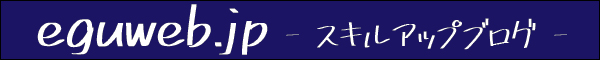# [Google Sheets] List of functions related to dates

We have compiled a list of functions related to “date” in Google Sheets!

## NETWORKDAYS.INTL

=NETWORKDAYS.INTL(start date, end date, [weekend], [holiday])

Returns the net number of workdays between two specified dates , excluding certain weekends and holidays . Only available in the new Google Sheets.

## DATE

=DATE(year, month, day)

Converts the specified year, month, and day to a date .

## DATE VALUE

=DATEVALUE(date string)

Converts a string of a given date in a known format to a serial number .

## DAY

=DAY(date)

Returns the day of the month corresponding to the specified date in numeric format.

## DAYS360

=DAYS360(start date, end date, method)

Returns the number of days between two dates , with 360 days in a year (used in interest rate calculations) .

Method – [Optional – Default is 0]
0 ​​is US style. If the start date is the last day of the month, the day of the start date is converted to 30 for calculation.
Other figures are European. If either the start date or the end date is the 31st, convert the date to 30.

## EDATE

=EDATE(start date, number of months)

Returns the date that is the specified number of months before or after the start date.

## EOMONTH

=EOMONTH(start date, number of months)

Returns the date of the last day of the month that is the specified number of months before or after the start date.

## HOUR

=HOUR(time)

Returns the hour argument for the specified time in numeric form.

## MINUTE

=MINUTE(time)

Returns the minute argument for the specified time in numeric form.

## MONTH

=MONTH(date)

Returns the month of the year corresponding to the specified date in numeric format.

## NETWORK DAYS

=NETWORKDAYS(start date, end date, holiday)

Returns the number of workdays contained between two specified dates.

## NOW

=NOW()

Returns a serial number corresponding to the current date and time.

## SECOND

=SECOND(time)

Returns the seconds argument for the specified time in numeric format.

## TIME

=TIME(hour, minute, second)

Converts the specified hour, minute, and second to time.

## TODAY

=TODAY()

Returns the serial number corresponding to the current date.

## WEEKDAY

=WEEKDAY(date, kind)

Returns the numeric day of the week corresponding to the specified date.

## YEAR

=YEAR(date)

Returns the year specified by the given date.

## DATEDIF

=DATEDIF(start date, end date, unit)

Calculates the number of days, months, and years between two dates.

## DAYS

=DAYS(end date, start date)

Returns the number of days between two dates.

## ISOWEEKNUM

=ISOWEEKNUM(date)

Returns the ISO week number of the year for the given date.

## TIME VALUE

=TIMEVALUE(time string)

Returns the percentage of the 24 hours in a day that the time represents. Only available in the new Google Sheets.

## WEEKNUM

=WEEKNUM(date, [type])

Returns a number representing the week number of the year in which the specified date falls. Only available in the new Google Sheets.

## WORKDAY

=WORKDAY(start_date, days, [holiday])

Calculates the end date based on the number of working days specified.

## YEAR FRAC

=YEARFRAC(start date, end date, [how to calculate days])

Returns the number of years (including partial years) between two dates using the specified number of days method.

Please refer to it ^ ^)en.eguweb.jp
Copied title and URL